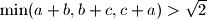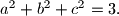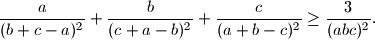IMO Shortlist 2011 problem A7

Kvaliteta:
Avg: 0,0
Težina:
Avg: 9,0
Let$a,b$ and$c$ be positive real numbers satisfying$\min(a+b,b+c,c+a) > \sqrt{2}$ and$a^2+b^2+c^2=3.$ Prove thatProposed by Titu Andrescu, Saudi Arabia
Izvor: Međunarodna matematička olimpijada, shortlist 2011